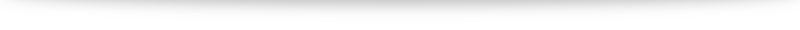# Alternative method to find food of perpendicular (without using vector projections)

In my video (link to be updated) I have worked out examples of how to use projection vectors to find the foot of perpendicular (for both lines and planes). The benefits of this method

1. it is faster,
2. it uses a similar concept for both lines and planes with slight modifications.

The downsides, however, is that it involves a rather unwieldy projection vector formula $(\mathbf{a}\cdot\mathbf{\hat{b}}) \mathbf{\hat{b}}$ and that minor details make a huge difference to whether we get the correct answer (e.g. where are our vectors pointing, $\overrightarrow{AB}$ or $\overrightarrow{BA}$? Is our final projection vector $\overrightarrow{AF}$ or $\overrightarrow{FB}$?).
In this post I will offer an alternative method to find foot of perpendicular using earlier concepts that some students find easier to understand.

### Finding foot of perpendicular to a line# Q9b discussion

### The integration part

We have $\displaystyle \int \frac{1}{(1-x^2)(1+x^2)} \; \mathrm{d}x = t + C$. Since the denominator is so complicated, partial fractions is the way to go.
$$\frac{1}{(1-x)(1+x)(1+x^2)} = \frac{A}{1-x} + \frac{B}{1+x} + \frac{Cx+D}{1+x^2}.$$
Give it a go!# Q6b discussion

Continuing from your method (making sure to use a new letter), we have $$\frac{a^2-b^2-2abi}{a+bi} = 0 + ic$$ Simplifying gives us $a^2-b^2 - 2abi = -bc + aci$. It looks very unwieldy, but let's use our usual techniques (comparing real and imaginary parts). Our final aim is to find $w$ in terms of $a$ so let's find $b$ and $c$ in terms of $a$. Give it a try first, and you can check against my working below:# Q3i discussion

Turns out for this question we don't need to use formulas like volume of hemisphere, etc.

Instead, we are given an equation of a curve $x^2 + (y-5)^2 = 25$. We want the volume of the water in the bowl. Able to spot which topic can help us tackle that?# Alternative method to find the line of intersection between two planes

In the video (link to be updated), we went through how to find the line of intersection between two planes using a GC. In this post, we will discuss an alternative method to accomplish this without the use of technology. Just like in our video, we will use the following example:
Find the equation of the line of intersection between $$p_1: \mathrm{r} \cdot \begin{pmatrix} 1 \\ 0 \\ 5 \end{pmatrix} = 7, \quad p_2: \mathrm{r} \cdot \begin{pmatrix} 1 \\ 1 \\ -1 \end{pmatrix} = -3.$$
The two important things we need to find an equation for a line are (1) a point on the line and (2) the direction vector parallel to the line. We will thus tackle the two separately:

#### Finding the direction vector

The key observation here is that the direction vector of the line of intersection is perpendicular to the normal vectors of both planes (try to visualize it!). Thus, to find the direction vector of the line of intersection, we can use the cross product. Hence